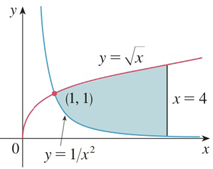Chapter 5.1, Problem 1E

Chapter
Section
Textbook Problem

# Find the area of the shaded region.To determine

To find:

The area of the shaded region.

Explanation

1) Concept:

The area A of the region bounded by the curves y=f(x), y=g(x) and the lines x=a and x=b, where f and g are continuous and f(x) g(x) for all x in [a, b]  is

A= abfx-gxdx

2) Given:

3) Calculation:

From the given graph,

we have y=x is the top (upper) boundary curve and y=1/x2 is the bottom (lower) boundary curve.

Let,  f(x)=x  and g(x)=1/x2

(1, 1) is the point of intersection of the given curves.

The shaded region lies between x=1 and x=4

Formula-

Area  A= abfx-gxdx

So the area of the shaded region is

A= 14x-1x2

### Still sussing out bartleby?

Check out a sample textbook solution.

See a sample solution

#### The Solution to Your Study Problems

Bartleby provides explanations to thousands of textbook problems written by our experts, many with advanced degrees!

Get Started

#### Find more solutions based on key concepts